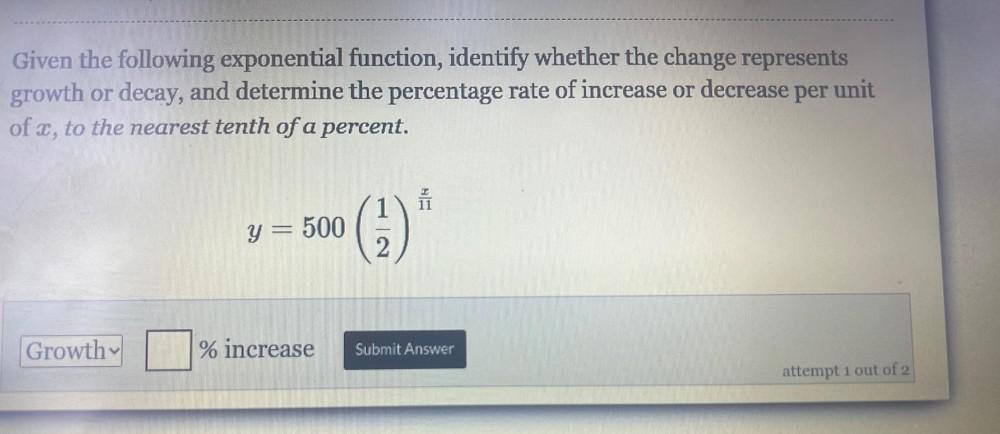Question:

# Given the following exponential function, identify whether the change represents growth or decay, and determine the percentage rate of increase or decrease per unit of x, to the nearest tenth of a perGiven the following exponential function, identify whether the change represents growth or decay, and determine the percentage rate of increase or decrease per unit of x, to the nearest tenth of a percent. y = 500(1/2)^(x/11)